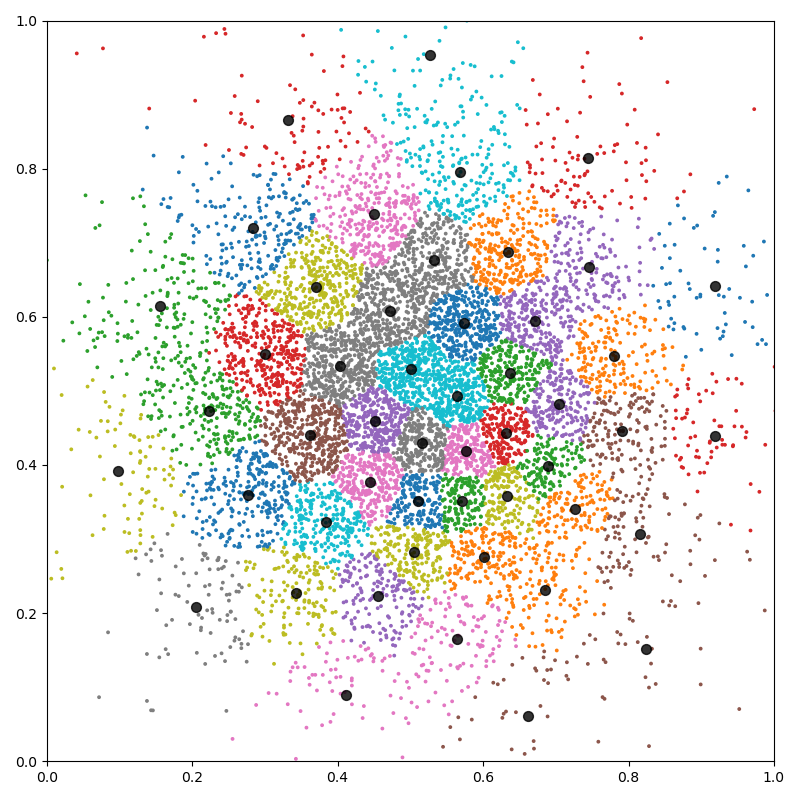# K-means clustering - NumPy API

The `pykeops.numpy.LazyTensor.argmin()` reduction supported by KeOps `pykeops.numpy.LazyTensor` allows us to perform bruteforce nearest neighbor search with four lines of code. It can thus be used to implement a large-scale K-means clustering, without memory overflows.

Note

For large and high dimensional datasets, this script is outperformed by its PyTorch counterpart which avoids transfers between CPU (host) and GPU (device) memories.

## Setup

Standard imports:

```import time

import numpy as np
from matplotlib import pyplot as plt

from pykeops.numpy import LazyTensor
import pykeops.config

dtype = "float32"  # May be 'float32' or 'float64'
```

Simple implementation of the K-means algorithm:

```def KMeans(x, K=10, Niter=10, verbose=True):
N, D = x.shape  # Number of samples, dimension of the ambient space

# K-means loop:
# - x  is the point cloud,
# - cl is the vector of class labels
# - c  is the cloud of cluster centroids
start = time.time()
c = np.copy(x[:K, :])  # Simplistic random initialization
x_i = LazyTensor(x[:, None, :])  # (Npoints, 1, D)

for i in range(Niter):

c_j = LazyTensor(c[None, :, :])  # (1, Nclusters, D)
D_ij = ((x_i - c_j) ** 2).sum(
-1
)  # (Npoints, Nclusters) symbolic matrix of squared distances
cl = D_ij.argmin(axis=1).astype(int).reshape(N)  # Points -> Nearest cluster

Ncl = np.bincount(cl).astype(dtype)  # Class weights
for d in range(D):  # Compute the cluster centroids with np.bincount:
c[:, d] = np.bincount(cl, weights=x[:, d]) / Ncl

end = time.time()

if verbose:
print(
"K-means example with {:,} points in dimension {:,}, K = {:,}:".format(
N, D, K
)
)
print(
"Timing for {} iterations: {:.5f}s = {} x {:.5f}s\n".format(
Niter, end - start, Niter, (end - start) / Niter
)
)

return cl, c
```

## K-means in 2D

First experiment with N=10,000 points in dimension D=2, with K=50 classes:

```N, D, K = 10000, 2, 50
```

Define our dataset:

```x = np.random.randn(N, D).astype(dtype) / 6 + 0.5
```

Perform the computation:

```cl, c = KMeans(x, K)
```

Out:

```K-means example with 10,000 points in dimension 2, K = 50:
Timing for 10 iterations: 0.02200s = 10 x 0.00220s
```

Fancy display:

```plt.figure(figsize=(8, 8))
plt.scatter(x[:, 0], x[:, 1], c=cl, s=30000 / len(x), cmap="tab10")
plt.scatter(c[:, 0], c[:, 1], c="black", s=50, alpha=0.8)
plt.axis([0, 1, 0, 1])
plt.tight_layout()
plt.show()
```## K-means in dimension 100

Second experiment with N=1,000,000 points in dimension D=100, with K=1,000 classes:

```if pykeops.config.gpu_available:
N, D, K = 1000000, 100, 1000
x = np.random.randn(N, D).astype(dtype)
cl, c = KMeans(x, K)
```

Out:

```K-means example with 1,000,000 points in dimension 100, K = 1,000:
Timing for 10 iterations: 5.52025s = 10 x 0.55202s
```

Total running time of the script: ( 0 minutes 7.644 seconds)

Gallery generated by Sphinx-Gallery